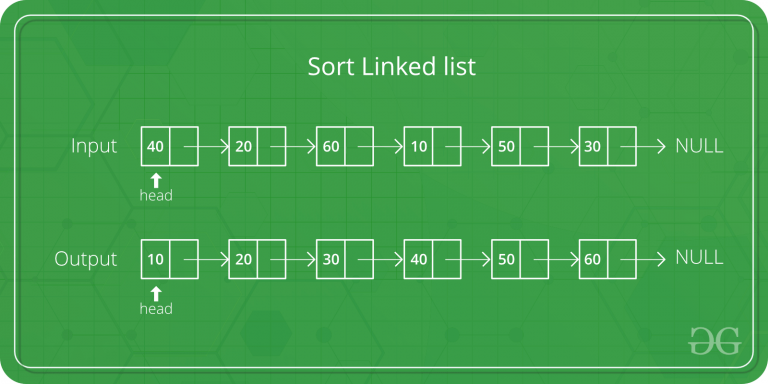# Python Program For Merge Sort Of Linked Lists

• Last Updated : 18 May, 2022

Merge sort is often preferred for sorting a linked list. The slow random-access performance of a linked list makes some other algorithms (such as quicksort) perform poorly, and others (such as heapsort) completely impossible.Let the head be the first node of the linked list to be sorted and headRef be the pointer to head. Note that we need a reference to head in MergeSort() as the below implementation changes next links to sort the linked lists (not data at the nodes), so the head node has to be changed if the data at the original head is not the smallest value in the linked list.

```MergeSort(headRef)
1) If the head is NULL or there is only one element in the Linked List
then return.
2) Else divide the linked list into two halves.
FrontBackSplit(head, &a, &b); /* a and b are two halves */
3) Sort the two halves a and b.
MergeSort(a);
MergeSort(b);
4) Merge the sorted a and b (using SortedMerge() discussed here)

## Python3

 `# Python3 program to merge sort of linked list`` ` `# create Node using class Node.``class` `Node:``    ``def` `__init__(``self``, data):``        ``self``.data ``=` `data``        ``self``.``next` `=` `None`` ` `class` `LinkedList:``    ``def` `__init__(``self``):``        ``self``.head ``=` `None``     ` `    ``# push new value to linked list``    ``# using append method``    ``def` `append(``self``, new_value):``         ` `        ``# Allocate new node``        ``new_node ``=` `Node(new_value)``         ` `        ``# if head is None, initialize it to new node``        ``if` `self``.head ``is` `None``:``            ``self``.head ``=` `new_node``            ``return``        ``curr_node ``=` `self``.head``        ``while` `curr_node.``next` `is` `not` `None``:``            ``curr_node ``=` `curr_node.``next``             ` `        ``# Append the new node at the end``        ``# of the linked list``        ``curr_node.``next` `=` `new_node``         ` `    ``def` `sortedMerge(``self``, a, b):``        ``result ``=` `None``         ` `        ``# Base cases``        ``if` `a ``=``=` `None``:``            ``return` `b``        ``if` `b ``=``=` `None``:``            ``return` `a``             ` `        ``# pick either a or b and recur..``        ``if` `a.data <``=` `b.data:``            ``result ``=` `a``            ``result.``next` `=` `self``.sortedMerge(a.``next``, b)``        ``else``:``            ``result ``=` `b``            ``result.``next` `=` `self``.sortedMerge(a, b.``next``)``        ``return` `result``     ` `    ``def` `mergeSort(``self``, h):``         ` `        ``# Base case if head is None``        ``if` `h ``=``=` `None` `or` `h.``next` `=``=` `None``:``            ``return` `h`` ` `        ``# get the middle of the list ``        ``middle ``=` `self``.getMiddle(h)``        ``nexttomiddle ``=` `middle.``next`` ` `        ``# set the next of middle node to None``        ``middle.``next` `=` `None`` ` `        ``# Apply mergeSort on left list ``        ``left ``=` `self``.mergeSort(h)``         ` `        ``# Apply mergeSort on right list``        ``right ``=` `self``.mergeSort(nexttomiddle)`` ` `        ``# Merge the left and right lists ``        ``sortedlist ``=` `self``.sortedMerge(left, right)``        ``return` `sortedlist``     ` `    ``# Utility function to get the middle ``    ``# of the linked list ``    ``def` `getMiddle(``self``, head):``        ``if` `(head ``=``=` `None``):``            ``return` `head`` ` `        ``slow ``=` `head``        ``fast ``=` `head`` ` `        ``while` `(fast.``next` `!``=` `None` `and` `               ``fast.``next``.``next` `!``=` `None``):``            ``slow ``=` `slow.``next``            ``fast ``=` `fast.``next``.``next``             ` `        ``return` `slow``         ` `# Utility function to print the linked list ``def` `printList(head):``    ``if` `head ``is` `None``:``        ``print``(``' '``)``        ``return``    ``curr_node ``=` `head``    ``while` `curr_node:``        ``print``(curr_node.data, end ``=` `" "``)``        ``curr_node ``=` `curr_node.``next``    ``print``(``' '``)``     ` `# Driver Code``if` `__name__ ``=``=` `'__main__'``:``    ``li ``=` `LinkedList()``     ` `    ``# Let us create a unsorted linked list``    ``# to test the functions created. ``    ``# The list shall be a: 2->3->20->5->10->15 ``    ``li.append(``15``)``    ``li.append(``10``)``    ``li.append(``5``)``    ``li.append(``20``)``    ``li.append(``3``)``    ``li.append(``2``)``     ` `    ``# Apply merge Sort ``    ``li.head ``=` `li.mergeSort(li.head)``    ``print` `(``"Sorted Linked List is:"``)``    ``printList(li.head)`` ` `# This code is contributed by Vikas Chitturi`

Output:

```Sorted Linked List is:
2 3 5 10 15 20```

Time Complexity: O(n*log n)

Space Complexity: O(n*log n)

Approach 2: This approach is simpler and uses log n space.

mergeSort():

1. If the size of the linked list is 1 then return the head
2. Find mid using The Tortoise and The Hare Approach
3. Store the next of mid in head2 i.e. the right sub-linked list.
4. Now Make the next midpoint null.
5. Recursively call mergeSort() on both left and right sub-linked list and store the new head of the left and right linked list.
6. Call merge() given the arguments new heads of left and right sub-linked lists and store the final head returned after merging.

1. Take a pointer say merged to store the merged list in it and store a dummy node in it.
2. Take a pointer temp and assign merge to it.
3. If the data of head1 is less than the data of head2, then, store head1 in next of temp & move head1 to the next of head1.
5. Move temp to the next of temp.
6. Repeat steps 3, 4 & 5 until head1 is not equal to null and head2 is not equal to null.
7. Now add any remaining nodes of the first or the second linked list to the merged linked list.
8. Return the next of merged(that will ignore the dummy and return the head of the final merged linked list)

## Python3

 `# Python program for the above approach`` ` ` ` `# Node Class``class` `Node:``     ` `    ``def` `__init__(``self``,key):``        ``self``.data``=``key``        ``self``.``next``=``None`` ` `# Function to merge sort``def` `mergeSort(head):``    ``if` `(head.``next` `=``=` `None``):``        ``return` `head``     ` `    ``mid ``=` `findMid(head)``    ``head2 ``=` `mid.``next``    ``mid.``next` `=` `None``    ``newHead1 ``=` `mergeSort(head)``    ``newHead2 ``=` `mergeSort(head2)``    ``finalHead ``=` `merge(newHead1, newHead2)``    ``return` `finalHead`` ` `# Function to merge two linked lists``def` `merge(head1,head2):``    ``merged ``=` `Node(``-``1``)``     ` `    ``temp ``=` `merged``    ``# While head1 is not null and head2``    ``# is not null``    ``while` `(head1 !``=` `None` `and` `head2 !``=` `None``):``        ``if` `(head1.data < head2.data):``            ``temp.``next` `=` `head1``            ``head1 ``=` `head1.``next``        ``else``:``            ``temp.``next` `=` `head2``            ``head2 ``=` `head2.``next``        ``temp ``=` `temp.``next``     ` `    ``# While head1 is not null``    ``while` `(head1 !``=` `None``):``        ``temp.``next` `=` `head1``        ``head1 ``=` `head1.``next``        ``temp ``=` `temp.``next``     ` `    ``# While head2 is not null``    ``while` `(head2 !``=` `None``):``        ``temp.``next` `=` `head2``        ``head2 ``=` `head2.``next``        ``temp ``=` `temp.``next``     ` `    ``return` `merged.``next`` ` `# Find mid using The Tortoise and The Hare approach``def` `findMid(head):``    ``slow ``=` `head``    ``fast ``=` `head.``next``    ``while` `(fast !``=` `None` `and` `fast.``next` `!``=` `None``):``        ``slow ``=` `slow.``next``        ``fast ``=` `fast.``next``.``next``    ``return` `slow`` ` `# Function to print list``def` `printList(head):``    ``while` `(head !``=` `None``):``        ``print``(head.data,end``=``" "``)``        ``head``=``head.``next`` ` `# Driver Code``head ``=` `Node(``7``)``temp ``=` `head``temp.``next` `=` `Node(``10``);``temp ``=` `temp.``next``;``temp.``next` `=` `Node(``5``);``temp ``=` `temp.``next``;``temp.``next` `=` `Node(``20``);``temp ``=` `temp.``next``;``temp.``next` `=` `Node(``3``);``temp ``=` `temp.``next``;``temp.``next` `=` `Node(``2``);``temp ``=` `temp.``next``;`` ` `# Apply merge Sort``head ``=` `mergeSort(head);``print``("``Sorted` `Linked ``List` `is``: ``");`` ` `printList(head);``  ` `# This code is contributed by avanitrachhadiya2155`

Output:

```Sorted Linked List is:
2 3 5 7 10 20 ```

Time Complexity: O(n*log n)

Space Complexity: O(log n)

Please refer complete article on Merge Sort for Linked Lists for more details!

My Personal Notes arrow_drop_up